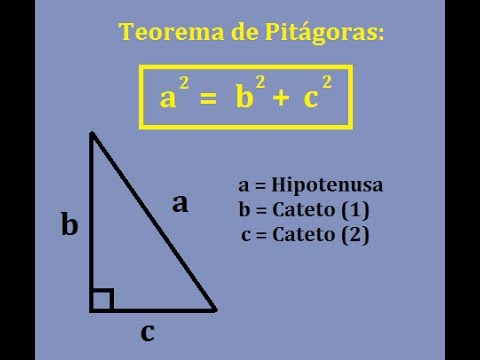# Pythagorean Theorem

The Pythagorean Theorem is one of the great advances in mathematics, a theory developed

by Pythagoras and the group who followed him, known as Pythagoreans. The popularity of the

Pythagorean Theorem is unmatched and today is considered one of the fundamental formulas

in geometry and mathematics in general. The simplicity with which it relates the different sides

of a right triangle opens the door for a huge number of applications that can be developed

based on this theorem.Pythagoras, with the help of his Pythagoreans, discovered that if we square each of the two

legs in a right triangle and add them together, the value will be the same as if we squared the

hypotenuse of the same triangle. We should remember that a leg is one of the sides that

“touches” the right angle of the triangle on one end; there are only two legs in every right

triangle and it is impossible for there to be just one or more than two as this would deform the

shape and no longer be a triangle. Likewise, the hypotenuse is the remaining side which is

always across from the right angle. We can also recognize the hypotenuse because of its size,

since it is the longest side of a right triangle.The discovery of the Pythagorean Theorem opened up a new world in mathematics known at

that time and even today it continues to surprise us and serve as a base for new theories that

help humanity delve deeper and deeper into a field as important as math. If you don’t know

the Pythagorean Theorem yet or if you are still having difficulties applying it, don’t worry; in

our examples section you can find many examples that can help answer your questions.